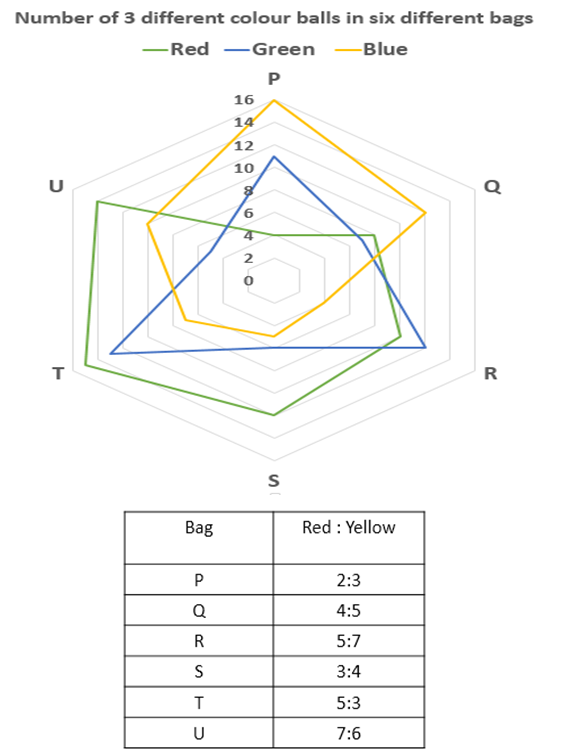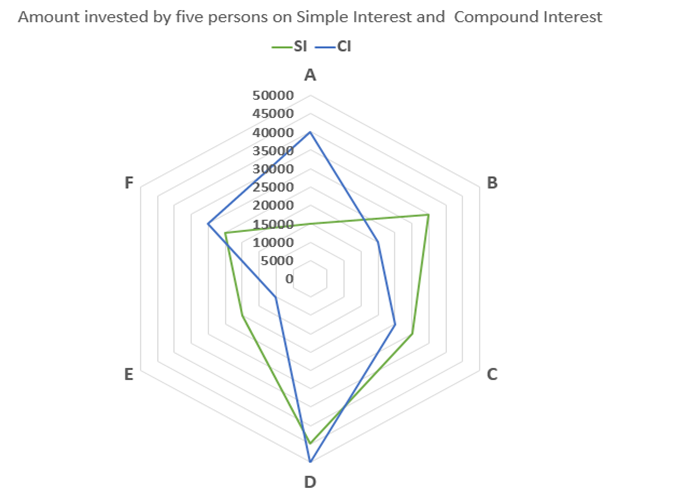# Quantitative Aptitude Questions (Data Interpretation) for SBI Clerk / IDBI Executive 2018 Day-46

Dear Readers, SBI is conducting Online preliminary Examination for the recruitment of Clerical Cadre. preliminary Examination of SBI Clerk was scheduled from June/July 2018. To enrich your preparation here we have providing new series of Data Interpretation – Quantitative Aptitude Questions. Candidates those who are appearing in SBI Clerk Prelims and IDBI Executive Exams can practice these Quantitative Aptitude average questions daily and make your preparation effective.

Click “Start Quiz” to attend these Questions and view Solutions

Directions (Q1 – 5): Study the following information carefully and answer the questions given below:Q1.  If four balls are drawn from bag Q, what is the probability that all the balls are of different colours?

a) 65/528

b) 53/527

c) 64/629

d) 53/523

e) None of these

Q2. Find the respective ratio of probabilities of drawing 2 red and 1 blue ball from bag R and from bag S.

a) 34547:9714

b) 27417:54340

c) 54340:27417

d) 22714:34547

e) None of these

Q3. Probability of drawing three green balls from bag T is what percent of the probability of drawing 3 yellow balls from bag U?

a) 104.63%

b) 106.43%

c) 103.46%

d) 104.36%

e) none of these

Q4. Total number of yellow balls in all the bags together is what percent more or less than the total number of green balls in all the bags together?

a) 27.04% less

b) 24.07% less

c) 27.04% more

d) 24.07% more

e) none of these

Q5. Find the product of the probabilities of drawing 2 green and 2 yellow balls from bag P and 2 red and 2 blue balls from bag U.

a) 45043/89158362

b) 45045/89158362

c) 45045/89178362

d) 45005/89178362

e) none of these

Directions (Q. 6 – 10): Study the following information carefully and answer the questions given below:1. Amount invested by A, B and C on SI is what percent of the amount invested by D, E and F on CI?

a) 82.28%

b) 84.48%

c) 87.78%

d) 88.88%

e) none of these

1. If A invested his amount on CI for 3 years at the rate of 15% per annum and D invested his amount on SI for 5 years at the rate of 18% per annum, find the respective ratio of total amount of A and D after 3 and 5 years respectively.

a) 16100 : 12175

b) 12175 : 16100

c) 12167 : 17100

d) 17100 : 12167

e) none of these

1. Simple interest earned by B after 10 years at the rate of 8% per annum is what percent more than the simple interest earned by F after 12 years at the rate of 5% per annum?

a) 86.67%

b) 68.68%

c) 86.76%

d) 68.86%

e) none of these

1. Find the sum of amounts earned on compound interest by B,C and D at the rate of 10% per annum after 2, 3 and 4 years respectively.

a) Rs.150680

b) Rs.130680

c) Rs.120680

d) Rs.140680

e) none of these

1. Sum of amounts invested by all the persons in simple interest is what percent more/less than the sum of amounts invested by all the persons in compound interest.

a) 2.94% less

b) 2.94% more

c) 2.85% less

d) 2.85% more

e) none of these

Directions (Q1 – 5):

In bag Q:

Red = 8

Green = 7

Blue = 12

Yellow = 5/4 x 8 = 10

Total = 37

Required probability =(8c1 x 7c1 x 12c1 x10c1)/37c4

= (8 x 7 x 12 x 10)/66045

= 64/629

In bag R:

Red = 10

Green = 12

Blue = 4

Yellow = 7/5 x 10 = 14

Total = 40

Probability of drawing 2 red and 1 blue ball from bag R = (10c2 x 4c1)/40c3

= (45 x 4)/9880

= 9/494

Now,

In bag S:

Red = 12

Green = 6

Blue = 5

Yellow = 4/3 x 12 = 16

Total = 39

Probability of drawing 2 red and 1 blue ball from bag S = (12c2 x 5c1)/39c3

= (66 x 5)/9139

= 330/9139

Required ratio = 9/494 : 330/9139 = 27417:54340

In bag T:

Red = 15

Green = 13

Blue = 7

Yellow = 3/5 x 15 = 9

Total = 44

Probability of drawing three green balls from bag T = 13c3/44c3

= 286/13244

= 13/602

In bag U:

Red = 14

Green = 5

Blue = 10

Yellow = 6/7 x 14 = 12

Total = 41

Probability of drawing three yellow balls from bag U = 12c3/41c3

= 220/10660

= 11/533

Required percentage = (13/602)/(11/533) x 100 = 104.63%

Total number of green balls in all the bags together = 11 + 7 + 12 + 6 + 13 + 5 = 54

Total number of yellow balls in all the bags together

= 3/2 x 4 + 5/4 x 8 + 7/5 x 10 + 4/3 x 12 + 3/5 x 15 + 6/7 x 14

= 6 + 10 + 14 + 16 + 9 + 12

= 67

Required percentage = (67 – 54)/54 x 100 = 24.07% more.

In bag P:

Red = 4

Green = 11

Blue = 16

Yellow = 3/2 x 4 = 6

Total = 37

Probability of drawing 2 green and 2 yellow balls from bag P = (11c2 x 6c2)/37c4

= (55 x 15)/66045

= 55/4403

In bag U:

Red = 14

Green = 5

Blue = 10

Yellow = 6/7 x 14 = 12

Total = 41

Probability of drawing 2 red and 2 blue balls from bag U = (14c2 x 10c2)41c4

= (91 x 45)/101270

= 819/20254

Required product = 55/4403 x 819/20254

= 45045/89178362

Directions (Q. 6 – 10):

Amount invested by A, B and C on SI = 15000 + 35000 + 30000 = 80000

Amount invested by D, E and F on CI = 50000 + 10000 + 30000 = 90000

Required percentage = (80000/90000) x 100 = 88.88%

Amount of A after three years = 40000 x 115/100 x 115/100 x 115/100 = Rs.60835

Amount of D after five years = (45000 x 18 x 5)/100 + 45000 = 40500 + 45000 = Rs.85500

Required ratio = 60835 : 85500 = 12167 : 17100

Simple interest earned by B after 10 years at the rate of 8% per annum = (35000 x 10 x 8)/100

= Rs.28000

Simple interest earned by F after 12 years at the rate of 5% per annum = (25000 x 12 x 5)/100

= Rs.15000

Required percentage = (28000 – 15000)/15000 x 100 = 86.67%

Amount earned by B on compound interest at the rate of 10% after 2 years

= 20000 x 110/100 x 110/100

= Rs.24200

Amount earned by C on compound interest at the rate of 10% after 3 years

= 25000 x 110/100 x 110/100 x 110/100

= Rs.33275

Amount earned by D on compound interest at the rate of 10% after 4 years

= 50000 x 110/100 x 110/100 x 110/100 x 110/100

= Rs.73205

Required sum = 24200 + 33275 + 73205 = Rs.130680

Sum of amounts invested by all the persons in simple interest

= 15000 + 35000 + 30000 + 45000 + 20000 + 25000

= 170000

Sum of amounts invested by all the persons in compound interest

= 40000 + 20000 + 25000 + 50000 + 10000 + 30000

= 175000

Required percentage = (175000 – 170000)/175000 x 100 = 2.85% less

Daily Practice Test Schedule | Good Luck

 Topic Daily Publishing Time Daily News Papers & Editorials 8.00 AM Current Affairs Quiz 9.00 AM Logical Reasoning 10.00 AM Quantitative Aptitude “20-20” 11.00 AM Vocabulary (Based on The Hindu) 12.00 PM Static GK Quiz 1.00 PM English Language “20-20” 2.00 PM Banking Awareness Quiz 3.00 PM Reasoning Puzzles & Seating 4.00 PM Daily Current Affairs Updates 5.00 PM Data Interpretation / Application Sums (Topic Wise) 6.00 PM Reasoning Ability “20-20” 7.00 PM English Language (New Pattern Questions) 8.00 PM General / Financial Awareness Quiz 9.00 PM